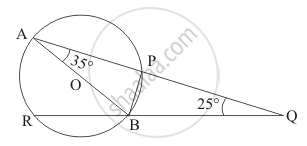Advertisement Remove all ads

# In the given figure, AB is a diameter of the circle such that ∠A = 35° and ∠Q = 25°, find ∠PBR. - Mathematics

Answer in Brief

In the given figure, AB is a diameter of the circle such that ∠A = 35° and ∠Q = 25°, find ∠PBR.Advertisement Remove all ads

#### Solution

Let us first consider the triangle ΔABQ.It is known that in a triangle the sum of all the interior angles add up to 180°.

So here in our triangle ΔABQ we have,

angleBAQ + angleAQB + angleABQ  = 180°

angleABQ = 180° - angleBAQ - angle AQB

= 180° - 35° - 25°

angle ABQ  = 120°

By a property of the circle we know that an angle formed in a semi-circle will be 90°..

In the given circle since ‘AB’ is the diameter of the circle the angle angleAPB  which is formed in a semi-circle will have to be 90°.

So, we have  angleAPB = 90°

Now considering the triangle  ΔAPB  we have,

angleAPB + angleBAP + angleABP  = 180°

angleABP  = 180° - angleAPB - angleBAP

= 180° - 90° - 35 °

angleABP  = 55°

From the given figure it can be seen that,

angleABP + anglePBQ = angle ABQ

anglePBQ = angleABQ - angleABP

= 120°- 55°

anglePBQ = 65°

Now, we can also say that,

anglePBQ + anglePBR = 180°

anglePBR = 180° - anglePBQ

=180° - 65 °

anglePBR   = 115°

Hence the measure of the angle  anglePBR  is 115°.

Is there an error in this question or solution?
Advertisement Remove all ads

#### APPEARS IN

RD Sharma Mathematics for Class 9
Chapter 15 Circles
Q 6 | Page 108
Advertisement Remove all ads

#### Video TutorialsVIEW ALL 

Advertisement Remove all ads
Share
Notifications

View all notifications

Forgot password?
Course